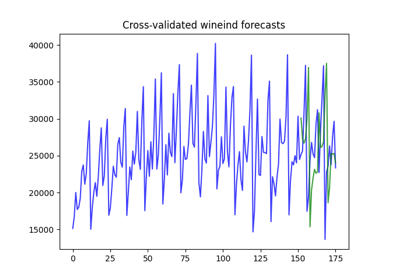# pmdarima.model_selection.cross_val_predict¶

pmdarima.model_selection.cross_val_predict(estimator, y, exogenous=None, cv=None, verbose=0, averaging='mean')[source][source]

Generate cross-validated estimates for each input data point

Parameters: estimator : estimator An estimator object that implements the fit method y : array-like or iterable, shape=(n_samples,) The time-series array. exogenous : array-like, shape=[n_obs, n_vars], optional (default=None) An optional 2-d array of exogenous variables. cv : BaseTSCrossValidator or None, optional (default=None) An instance of cross-validation. If None, will use a RollingForecastCV. Note that for cross-validation predictions, the CV step cannot exceed the CV horizon, or there will be a gap between fold predictions. verbose : integer, optional The verbosity level. averaging : str or callable, one of [“median”, “mean”] (default=”mean”) Unlike normal CV, time series CV might have different folds (windows) forecasting the same time step. After all forecast windows are made, we build a matrix of y x n_folds, populating each fold’s forecasts like so: nan nan nan # training samples nan nan nan nan nan nan nan nan nan 1 nan nan # test samples 4 3 nan 3 2.5 3.5 nan 6 5 nan nan 4  We then average each time step’s forecasts to end up with our final prediction results.

Examples

>>> import pmdarima as pm
>>> from pmdarima.model_selection import cross_val_predict,    ...     RollingForecastCV

## Examples using pmdarima.model_selection.cross_val_predict¶